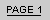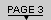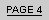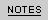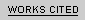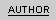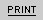I N   S E A R C H   O F   M A S C U L I N I T YP a g e   T h r e e :   T h e   F i n a l   S c e n e   o f   H u d " I   w o n ' t   b e   b a c k   t h i s   w a y , "   s a y s   L o n n i e   t o   H u d .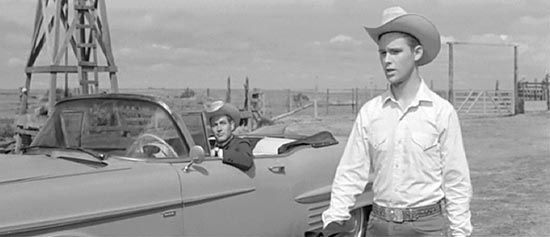A R T I C L E   B Y   A N N   B A R R O W